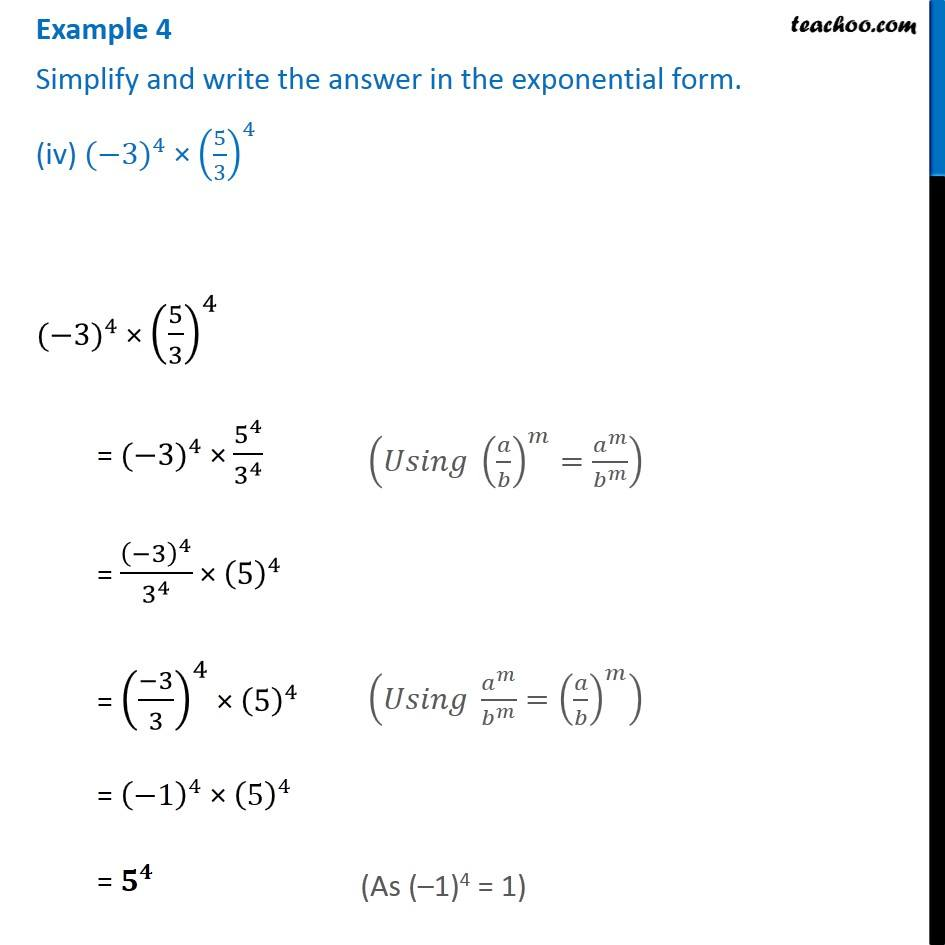Examples

Chapter 10 Class 8 Exponents and Powers
Serial order wiseLearn in your speed, with individual attention - Teachoo Maths 1-on-1 Class

### Transcript

Example 4 Simplify and write the answer in the exponential form. (iv) 〖(−3)〗^4 × (5/3)^4 〖(−3)〗^4 × (5/3)^4 = 〖(−3)〗^4 × 5^4/3^4 = (−3)^4/3^4 × (5)^4 = ((−3)/3)^4 × (5)^4 = (−1)^4 × (5)^4 = 𝟓^𝟒 (𝑈𝑠𝑖𝑛𝑔 (𝑎/𝑏)^𝑚=𝑎^𝑚/𝑏^𝑚 ) (𝑈𝑠𝑖𝑛𝑔 𝑎^𝑚/𝑏^𝑚 =(𝑎/𝑏)^𝑚 ) (As (–1)4 = 1)